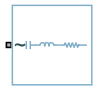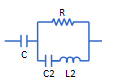# Passive Harmonic Filter (Three-Phase)

Harmonic current filter

• Library:
• Simscape / Electrical / Passive

•## Description

The Passive Harmonic Filter (Three-Phase) block suppresses system harmonic currents and decreases voltage distortion by providing low-impedance paths for the harmonics. At the rated frequency, the passive shunt filters are capacitive and provide reactive power, which can improve electrical power factor.

Each of the four models for the block corresponds to an option for the Filter type parameter:

### Band-Pass Filter, Single TunedAt the tuned frequency, LC resonance occurs and the impedance of the filter reaches its minimum, which equals the value of the resistance.

The filter tuned frequency is defined by this equation:

`${f}_{1}=n{f}_{0}=\frac{1}{2\pi \sqrt{LC}},$`

where f1 and f0 are the tuned and fundamental frequency, n is the harmonic order of the tuned frequency, L is the inductance and C is the capacitance.

The quality factor is defined as the ratio between the inductive or capacitive reactance at the tuned frequency and the resistance, as described by this equation:

`${Q}_{f}=\frac{{X}_{LN}}{R}=\frac{{X}_{CN}}{R},$`

where:

• R is the resistance.

• `XLN` is the impedance of the inductor at the tuned frequency ${X}_{LN}=2\pi {f}_{1}L.$

• `XCN` is the impedance of the capacitor at the tuned frequency ${X}_{CN}=\frac{1}{2\pi {f}_{1}C}.$

Higher quality factor values result in sharper frequencies. However, this produces high-power dissipation at the base frequency due to a relative low resistance.

The rated reactive power is given by:

`${Q}_{r}=\frac{{V}^{2}}{{X}_{L0}-{X}_{C0}}=\frac{{V}^{2}}{\frac{{X}_{C0}}{{n}^{2}}-{X}_{C0}}=\frac{{V}^{2}}{{X}_{C0}}\cdot \frac{{n}^{2}}{{n}^{2}-1},$`

where Qr is the rated reactive power for one phase and V is the branch voltage in root mean square.

### Band-Pass Filter, Double TunedA double-tuned filter has two tuned frequencies, f1 and f2, where ${f}_{1}={n}_{1}{f}_{0}$ and ${f}_{2}={n}_{2}{f}_{0}.$

The double-tuned filter comprises a series LC and a parallel RCL circuit, each tuned at frequencies fs and fp, close to the mean geometric frequency of f1 and f2, which are represented by the equation:

`${f}_{m}=\sqrt{{f}_{1}{f}_{2}}=\sqrt{{f}_{s}{f}_{p}},$`

where

`${f}_{s}=\frac{1}{2\pi \sqrt{LC}}$`

`${f}_{p}=\frac{1}{2\pi \sqrt{{L}_{2}{C}_{2}}}.$`

The quality factor of this filter is defined as the quality factor of the parallel `R` and `L` elements at the mean geometric frequency, fm:

`${Q}_{f}=\frac{R}{2\pi {f}_{m}{L}_{2}}.$`

### High-Pass Filter, Second-OrderThe second order high-pass filter shunts a large percentage of the harmonics at and above the tuned frequency. The filter is designed to have a flat impedance for high-order harmonics.

The tuned frequency is described by this equation:

`${f}_{1}=n{f}_{0}=\frac{1}{2\pi \sqrt{LC}}.$`

The quality factor is the reciprocal of the band-pass, single-tuned filter:

`${Q}_{f}=\frac{R}{{X}_{LN}}=\frac{R}{{X}_{CN}}.$`

The rated reactive power is the same of the band-pass, single-tuned filter:

`${Q}_{r}=\frac{{V}^{2}}{{X}_{L0}-{X}_{C0}}=\frac{{V}^{2}}{\frac{{X}_{C0}}{{n}^{2}}-{X}_{C0}}=\frac{{V}^{2}}{{X}_{C0}}\cdot \frac{{n}^{2}}{{n}^{2}-1}.$`

### High-Pass Filter, C-typeCompared to the single-tuned version, the C-type, high-pass filter has lower losses at the fundamental frequency, because the capacitor and inductor are parallel with the resistor.

To prevent fundamental currents from passing through the resistor, the resonance frequency of L2 and C2 is tuned to the fundamental frequency:

`${f}_{0}=\frac{1}{2\pi \sqrt{{L}_{2}{C}_{2}}}.$`

The quality factor is calculated using this equation:

`${Q}_{f}=\frac{R}{2\pi {f}_{0}n{L}_{2}}.$`

### Values of RCL Filter Components

Single-TunedDouble-TunedSecond-Order, High-PassC-type, High-Pass
R
`$\frac{1}{2\pi {f}_{0}C{Q}_{f}}$`
`$2\pi {f}_{m}{L}_{2}{Q}_{f}$`
`$2\pi n{f}_{0}L{Q}_{f}$`
`${Q}_{f}\left(2\pi \text{\hspace{0.17em}}n\text{\hspace{0.17em}}{f}_{0}\text{\hspace{0.17em}}{L}_{2}\right)$`
L
`$\frac{1}{C{\left(2\pi n{f}_{0}\right)}^{2}}$`
`$\frac{{V}^{2}}{{Q}_{r}\pi {f}_{0}\left({n}_{1}^{2}+{n}_{2}^{2}-2\right)}$`
`$\frac{1}{C{\left(2\pi n{f}_{0}\right)}^{2}}$`
None
C
`$\frac{{Q}_{r}}{2\pi {f}_{0}{V}^{2}}\cdot \frac{{n}^{2}-1}{{n}^{2}}$`
`$\frac{{Q}_{r}}{4\pi {f}_{0}{V}^{2}}\left(\frac{{n}_{1}^{2}-1}{{n}_{1}^{2}}+\frac{{n}_{2}^{2}-1}{{n}_{2}^{2}}\right)$`
`$\frac{{Q}_{r}}{2\pi {f}_{0}{V}^{2}}\cdot \frac{{n}^{2}-1}{{n}^{2}}$`
`$\frac{{Q}_{r}}{2\pi {f}_{0}{V}^{2}}$`
L2None
`$\begin{array}{c}\frac{\left(1-\frac{{f}_{1}^{2}}{{f}_{s}^{2}}\right)\left(1-\frac{{f}_{1}^{2}}{{f}_{p}^{2}}\right)}{C\left(2\pi {f}_{1}{}^{2}\right)}\\ where\text{\hspace{0.17em}}\text{\hspace{0.17em}}{f}_{s}=\frac{1}{2\pi \sqrt{LC}},\text{\hspace{0.17em}}{f}_{p}=\frac{{f}_{1}{f}_{2}}{{f}_{s}}\end{array}$`
None
`$\frac{1}{{C}_{2}{\left(2\pi n{f}_{0}\right)}^{2}}$`
C2None
`$\frac{1}{{L}_{2}{\left(2\pi {f}_{p}\right)}^{2}}$`
None
`$C\left({n}^{2}-1\right)$`

## Ports

### Conserving

expand all

Expandable three-phase port associated with the voltage.

## Parameters

expand all

Whether to model composite or expanded three-phase ports.

Composite three-phase ports represent three individual electrical conserving ports with a single block port. You can use composite three-phase ports to build models that correspond to single-line diagrams of three-phase electrical systems.

Expanded three-phase ports represent the individual phases of a three-phase system using three separate electrical conserving ports.

Reactive power at rated frequency and voltage, in V*A.

Rated phase-to-phase root mean squared (RMS) voltage, in V.

Fundamental frequency, in Hz.

Index of the sharpness of the tuned frequency.

Type of harmonic filter.

#### Dependencies

The Tuned frequency parameter becomes visible if you select `Band-pass filter, single-tuned`, `High-pass filter, second-order`, or `High-pass filter, C-type` for the Filter type parameter.

If you select ```Band-pass filter, double-tuned``` for the Filter type parameter, the Tuned frequency 1 and Tuned frequency 2 parameters become visible.

Type of connection

Tuned frequency for the filter, in Hz.

#### Dependencies

This parameter is visible only when you select ```Band-pass filter, single-tuned```, ```High-pass filter, second-order```, or ```High-pass filter, C-type``` for the Filter type parameter.

First tuned frequency, in Hz.

#### Dependencies

This parameter is visible only when you select ```Band-pass filter, double-tuned``` for the Filter type parameter.

Second tuned frequency, in Hz.

#### Dependencies

This parameter is visible only when you select ```Band-pass filter, double-tuned``` for the Filter type parameter.

## Version History

Introduced in R2019b